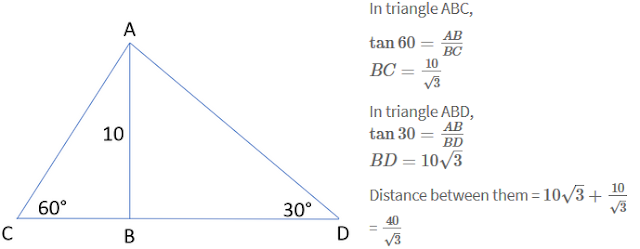Quantitative Aptitude Questions for SSC/Railway Exams (22 – 02 – 2018)

Quantitative Aptitude Questions for SSC/Railway Exams (22 – 02 – 2018)
1. There are 105 students in a class out of which 3/5th are boys, and remaining are girls. The number of boys is how much percentage more than the number of girls?
a) 58%
b) 46%
c) 72%
d) 50%
Explanation: Out of the 105 students, 3/5th are boys.
So, number of boys = 3 * 105 / 5 = 63
Number of girls = 105 - 63 = 42
So percentage by which boys are greater than girls can be given by = (63 – 42)/42 * 100
Required % = 2100/42 = 50%
2. A shopkeeper buys an article for a certain amount. He then sells the article for Rs. 30 more to a middleman. The middleman then marks up the price by 10 percent and then sells it to the customer. If the net increase in the price of the article (after 2 transactions) is Rs. 93, the cost price of the article is
a) Rs.500
b) Rs.400
c) Rs.300
d) Rs.600
Explanation: Let the cost price of the article be ‘c’.
The middleman buys the article for c+30 rupees.
He sells the article for 1.1*(c+30) = 1.1c + 33 rupees.
We know that the net increase in the price of the article is Rs. 93
1.1c + 33 - c = Rs. 93
0.1c = Rs.60
c = Rs. 600
3. Find the HCF of 2/3, 1/2, 3/5
a) 6
b) 1/30
c) 1/15
d) 1/10
Explanation: HCF = HCF of numerators/ LCM of denominators
HCF of 1, 2, 3 = 1
LCM of 3, 2, 5 = 30

4. Mohit can do a particular work in 12 hours. Mohit and Sumit can together finish the same work in 6 hours and 40 minutes. How much time will Sumit take to finish the work alone?
a) 10 hours
b) 9 hours
c) 15 hours
d) 12 hours
Explanation: Let Sumit alone finishes the work in ‘x’ hours.
We know that 6 hours 40 minutes can be written as 6 2/3 = 20/3 hours
Work done by Mohit in 1 hour = 1/12 * total work
Work done by Sumit in 1 hour = 1/x * total work
Total work done in 1 hour = 3/20 * total work
So we have
1/12 + 1/x = 3/20 => 1/x = 3/20 – 1/12 = (9 – 5)/60 = 4/60 = 1/15 => x = 15
Hence, Sumit alone takes 15 hours to finish the work.
5. Two friends are standing in a straight line with a pole of height 10m in between them. They find the angle of elevation of the top of the pole to be  30° and 60° respectively. Find the distance between the two friends.
a) 10 (1 + √3)
b) 30 (√3 + 1)
c) 10√3
d) 40/√3
Explanation:6. Which of the following option have fractions arranged in increasing order of their value?
a) 2/6, 1/5, 3/8, 4/7, 5/6
b) 1/5, 3/8, 2/6, 4/7, 5/6
c) 1/5, 2/6, 3/8, 4/7, 5/6
d) 2/6, 1/5, 4/7, 3/8, 5/6
Explanation: 1/5 = 0.2; 2/6 = 0.33; 3/8 = 0.375; 4/7 = 0.57; 5/6 = 0.83
7. If x * (x + 16) + 64 = 0 and y * (y – 6) + 9 = 0. What is the value of x^2 + xy + y^2?
a) 49
b) 38
c) 53
d) 61
Explanation:8. A cow is tied to one of the corners of a square-shaped field of side 10 m. The length of the rope is 7 m. Find the area of the field that the cow cannot graze.
a) 45.8 sq.m
b) 61.5 sq.m
c) 75 sq.m
d) 50 sq.m
Explanation: The cow is tied to one corner of the field. The length of the rope is 7m. Hence, the cow can graze an area equal to the sector of radius 7m and central angle 90°.
The area of the sector = 90/360 * П * 7^2 = 38.5
The area of the field = 10^2 = 100
Hence, the area which the cow cannot graze = 100 - 38.5 = 61.5 sq. m

9. If cosec(30°) + cot(60°) = y. What is the value of y?
a) (2√3 + 1)/√3
b) (√3 + 1)/√3
c) (3√2 + 1)/√2
d) (2√2 + 1)/√2